# Measurement of evaporation residue cross-sections of the reaction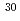Si +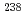U at subbarrier energies
Seaborgium isotopes were produced in the fusion reactionSi +U as evaporation residues (ERs), and the cross sections were determined. The experiment was carried out at GSI in Darmstadt, Germany. At the center-of-mass energy of E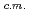= 144 MeV, three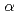decay chains starting from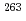Sg were observed, and the corresponding ER cross section was determined to be 67 pb. At the sub-barrier energy of E= 133 MeV, three spontaneous fission events of a new isotopeSg were detected. The cross section was 10 pb. The half-life ofSg was determined to be 120 ms. The ER cross sections were compared with a statistical model calculation. In the fusion process, the coupled channel calculation taking into account the prolate deformation ofU was adopted to determine the capture cross section. The calculated capture cross section agrees well with the fission cross section ofSi +U obtained at the JAEA tandem accelerator. The measured cross section ofSg at the sub-barrier energy is factor 10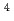larger than the calculation based on the one-dimensional model in the fusion process, showing the fusion enhancement caused by the deformation ofU. However, disagreement with the calculation suggests the presence of quasi-fission channel. At the above barrier energy of E= 144 MeV, the measured cross section is well reproduced by the calculation. This means that the interaction ofSi at the equotorial side ofU has advantage on the fusion process.